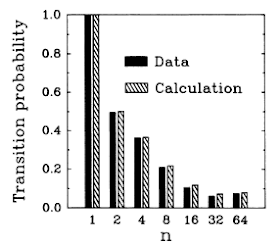via Filozofy
The standard axioms of quantum mechanics imply that in the limit of continuous observation a quantum system cannot evolve.
(Andrew Hodges in Alan Turing: the logical and physical basis of computing - pdf)
Initially known as Turing’s paradox, in honor of the mathematician who formulated it in the 1950s, was subsequently identified as quantum Zeno effect, resulting an advanced version of the famous Zeno’s arrow paradox, whose phylosophical result is the negation of motion. A first formulation and derivation of the effect is found in Does the lifetime of an unstable system depend on the measuring apparatus?(1), while George Sudarshan and Baidyanath Misra were the first to identify it as quantum Zeno paradox. The two theoretical physicists established that an unstable particle will not decay as long as it is kept under continuous observation(2). However they try to save goat and cabbage:
There is a fundamental principle in quantum theory that denies the possibility of continuous observation.(2)
On the other hand, Ghirardi, Omero, Weber and Rimini show that:
if the uncertainty relations are properly taken into account the arguments leading to the paradox are not valid.(3)
The key role in the demonstration of Ghirardi et al. is the time and energy reformulation of the Heisenberg’s uncertainty principle(4): $\Delta H \cdot \Delta T \leq \frac{\hbar}{2}$ If we want measure more frequently (up to the continuous), we nust necessarily reduce the time for the measure itself, and this leads to larger energies(3).
These objections, however, didn’t prevent the theorists to speculate about this quantum formulation of the arrow paradox: Richard Cook, for example, suggested an experiment(5) that should demonstrate the reality of the paradox. The experiment, which involved the capture of an ion, was actually carried out in 1989 by NIST(6) using about 5000 ions of $^9 Be^+$ and thus demonstrating the reality of the effect:Transition $1 \rightarrow 2$Transition $2 \rightarrow 1$
The results, as always in science, instead of ending the story, have opened new issues (also for the opposite effect, the quantum anti-Zeno effect, where continuous observation generates a probability of 1). Certainly there is to understand the link between the quantum Zeno effect and Heisenberg’s uncertainty principle for time and energy.
Another issue is to understand the validity of the effect: the paradox provides the possibility of instantaneous measurements, which is practically impossible. It is therefore necessary to understand the experimental conditions for which the effect should be presented: it is the target of the very logn paper by Koshino and Shimizu. They use quantum theory of measure to clarify a paradox that seems an effect(7).
One of the most recently result about the quantum Zeno paradox is dued by Paolo Facchi and Marilena Ligabò, that studied the effect over long periods(8). In particular, they focused on the mathematical behavior of the quantum probability $p^{(N)} (t)$, when both the time $t$ and the number of measurements $N$ go to infinity.
The two researchers have observed that the value of $p$ depends on a real parameter $\alpha$: for $0 \leq \alpha \leq 1/2$, the system is frozen in its initial state and the QZE takes place; for $1/2 < \alpha < 1$, the system has a classic behavior; for $\alpha = 1/2$, the probability has a gaussian behavior, $e{-t2 / \tau^2}$, where $\tau \propto \hbar^{-2}$.
Finally
if $\alpha \geq 1$ the limit probability is a strange beast and becomes sensitive to the spectral properties of the state $\psi$(8)
From a physical point of view, this fact complicates the situation and makes necessary further analysis. However, it is interesting to note that this effect may also have a long-term effect on quantum systems.
However, beyond the physical and philosophical arguments, it is very interesting to observe how the quantum Zeno effect seems to be a perfect counterbalance of adiabatic invariants, where non-observations play a key role: it seems that the two theorems counterbalance each other to create a perfect equilibrium within which any imperfect physical system evolves.
About Turing and physics, read also, always by Hodges, The Nature of Turing and the Physical World
1. Degasperis, A., Fonda, L., & Ghirardi, G. C. (1974). Does the lifetime of an unstable system depend on the measuring apparatus?. Il Nuovo Cimento A (1971-1996), 21(3), 471-484. doi:10.1007/BF02731351 (sci-hub)
2. Misra B. & Sudarshan ECG (1977). The Zeno’s Paradox in Quantum Theory, Journal of Mathematical Physics, 18 (4) 756. doi:10.1063/1.523304 (sci-hub)
3. Ghirardi GC, Omero C., Weber T. & Rimini A. (1979). Small-time behavior of quantum nondecay probability and Zeno’s paradox in quantum mechanics, Il Nuovo Cimento A A, 52 (4) 421-442. doi:10.1007/BF02770851 (pdf)
4. Mandelstam L. & Tamm I. (1945). The Uncertainty Relationship between Energy and Time in Non-Relativistic Quantum Mechanics, Journal of Physics-USSR, 9 (4) 115-123. doi:10.1007/978-3-642-74626-0_8 (pdf)
5. Cook RJ (1988). What are Quantum Jumps?, Physica Script, T21 49-51. doi:10.1088/0031-8949/1988/T21/009. See also
Cook R. & Kimble H. (1985). Possibility of Direct Observation of Quantum Jumps, Physical Review Letters, 54 (10) 1023-1026. doi:10.1103/PhysRevLett.54.1023 (pdf)
6. Itano W., Heinzen D., Bollinger J. & Wineland D. (1990). Quantum Zeno Effect, Physical Review A, 41 (5) 2295-2300. doi:10.1103/PhysRevA.41.2295 (pdf)
7. Koshino K. & Shimizu A. (2005). Quantum Zeno Effect by General Measurements, Physics Reports, 412 (4) 191-275. doi:10.1016/j.physrep.2005.03.001 (arXiv)
8. Facchi, P., & Ligabò, M. (2017). Large-time limit of the quantum Zeno effect. Journal of Mathematical Physics, 58 (3) doi:10.1063/1.4978851 (arXiv)